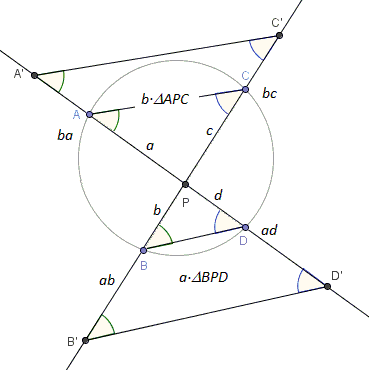# Intersecting Chords Theorem - a Visual Proof

Given a point $P$ in the interior of a circle, pass two lines through $P$ that intersect the circle in points $A$ and $D$ and, respectively, $B$ and $C$. Then $AP\cdot DP = BP\cdot CP$.If $a=AP$, $b=BP$, $c=CP$, and $d=DP$, then the Intersecting Chors Theorem is expressed as

$a\cdot d = b\cdot c$.

(The applet below illustrates the proof. Points $A$, $B$, $C$, $D$, $O$, $R$ are draggable. Point $O$ is the center of the given circle, $R$ - a point on the circle.)

### Proof

This proof is a slightly modified version of the one suggested by Hubert Shutrick.

Apply homothety with center $P$ and the coefficient $b$ to $\Delta APC$ and another with the coefficient $a$ to $\Delta BPD$.Observe that, as two inscribed angles subtended by the same arc, $\angle CAD =\angle CBD$, and, similarly, $\angle ACB =\angle ADB$. Since homotheties preserves angles, we also have $\angle C'A'D' =\angle C'B'D'$ and $\angle A'C'B' =\angle A'D'B'$. By the construction, $A'P = ba = ab = B'P$, making triangles $A'PC'$ and $B'PD'$ equal, so that $C'P=D'P$ but $C'P=bc$ whereas $D'P=ad$. Therefore, $bc=ad$.### Power of a Point wrt a Circle